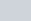A solid is composed of a cylinder with hemispherical ends.
Question:

A solid is composed of a cylinder with hemispherical ends. If the whole length of the solid is 104 cm and the radius of each of the hemispherical ends is 7 cm, find the cost of polishing its surface at the rate of Rs 10 per dm2 .

Solution:

We have a solid composed of cylinder with hemispherical ends.

Radius of the two curved surfaces $(r)=7 \mathrm{~cm}$

Height of cylinder is $h$.

Total height of the body $(h+2 r)=104 \mathrm{~cm}$

So, total surface area is given by,

Total surface area $=$ Curved surface area of cylinder $+$

$+2$ (Curves surface area of hemisphere)

$=2 \pi r h+2\left(2 \pi r^{2}\right)$

$=2 \pi r(h+2 r)$

$=2(3.14)(7)(104) \mathrm{cm}^{2}$

$=4571.84 \mathrm{~cm}^{2}$

Change the units of curved surface area as,

Total surface area $=\frac{4571.84}{100} \mathrm{dm}^{2}$

$=45.7184 \mathrm{dm}^{2}$

Cost of polishing the surface is Rs 10 per.

So total cost,

$=\operatorname{Rs}(45.7184)(10)$

$=$ Rs 457.18